Courses

# NCERT Exemplar - Isolation of Elements JEE Notes | EduRev

## JEE Revision Notes

Created by: Mohit Rajpoot

## JEE : NCERT Exemplar - Isolation of Elements JEE Notes | EduRev

The document NCERT Exemplar - Isolation of Elements JEE Notes | EduRev is a part of the JEE Course JEE Revision Notes.
All you need of JEE at this link: JEE

MULTIPLE CHOICE QUESTIONS (TYPE - I)

Q.1. In the extraction of chlorine by electrolysis of brine ____________.
(i) Oxidation of Cl ion to chlorine gas occurs.
(ii) Reduction of Cl ion to chlorine gas occurs.
(iii) For overall reaction ∆G° has negative value.
(iv) A displacement reaction takes place.
Ans. (i)
Solution.
oxidation of Cl- ion to chlorine gas occurs Following reaction takes place in the extraction of chlorine by electrolysis
2H2O(l) + 2H2O(l) 2Cl- (aq) → H2(g) + Cl2(g) + 2OH- (aq)
From the above reaction it is very clear that the oxidation of Cl- ion to chlorine gas occurs. Thus, option (i) is the correct answer.
∆°G for this reaction is +422kJ. Therefore, thermodynamically reaction is not feasible.
We know that
∆°G = -nFE°
By using this equation value of E° can be calculated. Thus,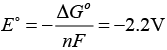Therefore, for the occurrence of this reaction, external potential (emf) greater than 2.2V is required. Due to this fact (iii) is not the correct option.

Q.2. When copper ore is mixed with silica, in a reverberatory furnace copper matte is produced. The copper matte contains ____________.
(i) Sulphides of copper (II) and iron (II)
(ii) Sulphides of copper (II) and iron (III)
(iii) Sulphides of copper (I) and iron (II)
(iv) Sulphides of copper (I) and iron (III)
Ans. (iii)
Solution.

When copper ore is mixed with sillica in a reverberatory furnace copper matte is produced. The copper matte contains sulphide of copper (I) and iron (II).
Copper matte → Cu2S and FeS

Q.3. Which of the following reactions is an example of autoreduction?
(i) Fe3O4 + 4CO → 3Fe + 4CO2
(ii) Cu2O + C → 2Cu + CO
(iii) Cu2+ (aq) + Fe (s) → Cu (s) + Fe2+ (aq)
(iv) Cu2O + 1/2 Cu2S →  3Cu + 1/2 SO2
Ans. (iv)
Solution.
This reaction includes reduction of copper (I) oxide by copper (I) sulphide. In this process, copper is reduced by itself hence this process is known as autoreduction and the solidified copper so obtained is known as blister copper.

Q.4. A number of elements are available in earth’s crust but most abundant elements are ____________.
(i) Al and Fe
(ii) Al and Cu
(iii) Fe and Cu
(iv) Cu and Ag

Ans. (i)
Solution.

Among a number of elements which are available in earth crust, the most abundant elements are aluminum and iron. Aluminium is third most abundant element in earth crust. i.e., 8.3% by weight while iron present in earth crust with 4.2% by weight. Copper and silver are also found in earth crust but their abundance percentage is low.

Q.5. Zone refining is based on the principle that ___________.
(i) Impurities of low boiling metals can be separated by distillation.
(ii) Impurities are more soluble in molten metal than in solid metal.
(iii) Different components of a mixture are differently adsorbed on an adosrbent.
(iv) Vapours of volatile compound can be decomposed in pure metal.
Ans. (ii)
Solution.

Zone refining is based on the principal that the impurities are more soluble in molten state than in solid state of the metal. A circular mobile heater is fixed at one end of impure metal rod. The molten zone moves along with heater which is moved forward.
As the heater moves forward, the pure metal crystallises out of the melt and the impurities pass on into adjacent molten zone.
At one end, impurities get concentrated. Metals like germanium, silicon, gallium, etc., are refined by this method.

Q.6. In the extraction of copper from its sulphide ore, the metal is formed by the reduction of Cu2O with
(i) FeS
(ii) CO
(iii) Cu2S
(iv) SO2
Ans. (iii)
Solution.

In the extraction of copper from its sulphide ore, the metal is formed by the reduction of Cu2O with Cu2S. This reaction completes with the process of autoreduction.
Chemical reaction involved here is as follows: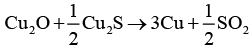In this process, copper appears as blister copper.

Q.7. Brine is electrolysed by using inert electrodes. The reaction at anode is ________.
(i) Cl (aq.) → 1/2 Cl(g) + e ; E°Cell V = 1.36V
(ii) 2H2O (l ) → O2 (g) + 4H+ + 4e ; E°Cell = 1.23V
(iii) Na+ (aq.) + e → Na(s) ; E°Cell V = 2.71V
(iv) H+ (aq.) + e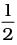H2 (g) ;  E°Cell V = 0.00V
Ans. (i)
Solution.

Brine is electrolysed by using inert electrodes. The possible reactions occurring at anode are
Cl- (aq) → 1/2 Cl2(g) + e-; E°Cell = 1.36V
2H2O(l) → O2 (g) + 4H+ + 4e- ; E°Cell = 1.23V
The reaction at anode with lower value of E° is preferred and therefore water should get oxidised in preference to Cl (aq). However, Cl2 is produced instead of O2. This unexpected result is explained on the basis of the fact that water needs greater voltage for oxidation to O2 (as it is kinetically slow process) than that needed for oxidation of Cl-ions toCl2

Q.8. In the metallurgy of aluminium ________________.
(i) Al3+ is oxidised to Al (s).
(ii) Graphide anode is oxidised to carbon monoxide and carbon dioxide.
(iii) Oxidation state of oxygen changes in the reaction at anode.
(iv) Oxidation state of oxygen changes in the overall reaction involved in the process.
Ans. (ii)
Solution.

graphide anode is oxidised to carbon monoxide and carbon dioxide.
In the metallurgy of aluminium, electrolysis is performed in a steel vessel with lining of carbon acts as cathode and graphite acts as anode. During this process graphite anode is oxidised to CO and CO2.
Chemical reaction occurring in this process is as follows
2 Al2O3 + 3C → 4Al + 3CO2
This process is known as Hall - Heroult process. The electrolytic reactions are
At cathode Al3+ (melt) + 3e− → Al (l)
At anode C(s) +O2− (melt) → CO (g) + 2e-
C(s) + 2O2-(melt) → CO2 (g) + 4e-

Q.9. Electrolytic refining is used to purify which of the following metals?
(i) Cu and Zn
(ii) Ge and Si
(iii) Zr and Ti
(iv) Zn and Hg
Ans. (i)
Solution.

Copper and zinc are two metals which are generally purified by using electrolyte refining. In this process, impure metal is used as anode and pure metal is used as a cathode. Impurities from the blister copper or impure zinc deposit as anode mud.

Q.10. Extraction of gold and silver involves leaching the metal with CN ion.  The metal is recovered by __________.
(i) Displacement of metal by some other metal from the complex ion.
(ii) Roasting of metal complex.
(iii) Calcination followed by roasting
(iv) Thermal decomposition of metal complex.
Ans. (i)
Solution.

Extraction of gold and silver involves leaching the metal with CN ion. The metal is recovered by displacement of metal by some other metal from the complex ion. This is an oxidation reaction.
4Au(s) + 8 CN- (aq) + 2H2O (aq) + O2 (g) → 4 [Au (CN) 2]- (aq) + 4 OH- (aq)
4 [Au (CN)2] (aq) + Zn (s) → 2 Au (s)+ [Zn (CN)4]2-(aq)
Here, Zn acts as a reducing agent.

Note : Answer the questions 11-13 on the basis of Fig. 6.1.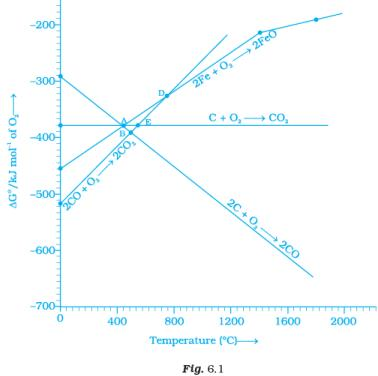Q.11. Choose the correct option of temperature at which carbon reduces FeO to iron and produces CO.
(i) Below temperature at point A.
(ii) Approximately at the temperature corresponding to point A.
(iii) Above temperature at point A but below temperature at point D.
(iv) Above temperature at point A.
Ans. (iv)
Solution.

At temperatures above point A, the C, CO line comes below the Fe, FeO line
ΔfGo (c,co) < ΔfGo(Fe,FeO)
Therefore, above point A, C reduces FeO to Fe forming carbon monoxide. Hence, reduction of FeO by carbon occurs above point A only.

Q.12. Below point ‘A’ FeO can ______________.
(i) Be reduced by carbon monoxide only.
(ii) Be reduced by both carbon monoxide and carbon.
(iii) Be reduced by carbon only.
(iv) Not be reduced by both carbon and carbon monoxide.
Ans. (i)
Solution.

Below point A, Gibbs free energy change for the formation of CO2 from CO has lower value than Gibb’s free energy change for the formation of FeO. Hence, FeO Will be reduced by CO only below point A.

Q.13. For the reduction of FeO at the temperature corresponding to point D, which of the following statements is correct?
(i) ∆G value for the overall reduction reaction with carbon monoxide is zero.
(ii) ∆G value for the overall reduction reaction with a mixture of 1 mol carbon and 1 mol oxygen is positive.
(iii) ∆G value for the overall reduction reaction with a mixture of 2 mol carbon and 1 mol oxygen will be positive.
(iv) ∆G value for the overall reduction reaction with carbon monoxide is negative.
Ans. (i)
Solution.

At point D, ∆G curve for formation of CO2 from CO and FeO from Fe intersect each other. Therefore, overall reduction of FeO with CO is zero Hence, (i) is the correct choice.

MULTIPLE CHOICE QUESTIONS (TYPE - II)

Note : In the following questions two or more options may be correct.
Q.14. At the temperature corresponding to which of the points in Fig.6.1, FeO will be reduced to Fe by coupling the reaction 2FeO → 2Fe + O2 with all of the following reactions?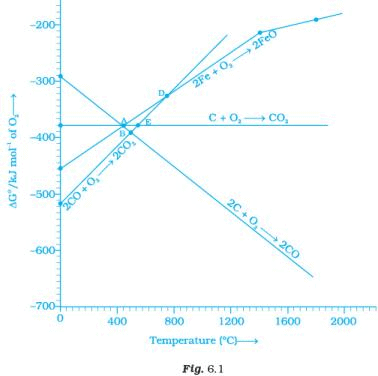(a) C + O2 → CO2
(b) 2C + O2 → 2CO  and
(c)  2CO + O2 → 2CO2
(i) Point A
(ii) Point B
(iii) Point D
(iv) Point E
Ans. (ii, iv)
Solution.

Below point B and E, FeO will be reduced to Fe by all the three reactions shown above in the question as ΔGo(c,co2), ΔGo(c,co), ΔGo(co,co2) lie below DG°(Fe,FeO) curve at point B and E.

Q.15. Which of the following options are correct?
(i) Cast iron is obtained by remelting pig iron with scrap iron and coke using hot air blast.
(ii) In extraction of silver, silver is extracted as cationic complex.
(iii) Nickel is purified by zone refining.
(iv) Zr and Ti are purified by van Arkel method.

Ans. (i, iv)
Solution.
Correct statements are
(i) Cast iron is obtained by remelting pig iron with scarp iron and coke using hot air blast.
(iv) Zr and Ti are purified by van Arkel method as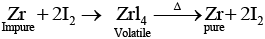(ii) and (iii) can be correctly stated as
(b) In extraction of silver, silver is extracted as anionic complex [Ag (CN)2]
(c) Nickel is purified by vapour phase refining method.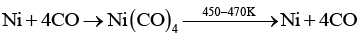Q.16. In the extraction of aluminium by Hall-Heroult process, purified Al2O3 is mixed with CaF2 to
(i) Lower the melting point of Al2O3.
(ii) Increase the conductivity of molten mixture.
(iii) Reduce Al3+ into Al(s).
(iv) Acts as catalyst.
Ans. (i, ii)
Solution.
In the metallurgy of aluminum Al2O3 is mixed with Na3AIF6 and CaF2 which causes following affects.
(i) Lower the melting point of Al2O3
(ii) Increase the conductivity of molten mixture

Q.17. Which of the following statements is correct about the role of substances added in the froth floation process?
(i) Collectors enhance the non-wettability of the mineral particles.
(ii) Collectors enhance the wettability of gangue particles.
(iii) By using depressants in the process two sulphide ores can be separated.
(iv) Froth stabilisers decrease wettability of gangue.
Ans. (i, iii)
Solution.
Froth floatation process is used to extract metal from sulphide ore. This method utilises collectors and depressants whose functions are as follows:
(i) Collectors enhance the non-wettability of the mineral particles.
(iii) By using depressants in the process two sulphide ores can be separated, e.g., sodium cyanide is used as a depressant to separate lead sulphide ore from zinc sulphide ore.

Q.18. In the Froth Floatation process, zinc sulphide and lead sulphide can be separated by ______________.
(i) Using collectors.
(ii) Adjusting the proportion of oil to water.
(iii) Using depressant.
(iv) Using froth stabilisers.
Ans. (ii, iii)
Solution.
Froth floatation method is used to extract metal from sulphide ore. ZnS and PbS can be Separated by using depressant and adjusting the proportion of oil to water. Depressant sec for this purpose is NaCN. It selectively prevents ZnS from coming to the froth. Hende, (ii) and (iii) are correct choices.

Q.19. Common impurities present in bauxite are _________.
(i) CuO
(ii) ZnO
(iii) Fe2O3
(iv) SiO2
Ans. (iii, iv)
Solution.
Bauxite is an ore of aluminum which contain Fe2O3 and SiO2 as common impurities.

Q.20. Which of the following ores are concentrated by froth floation?
(i) Haematite
(ii) Galena
(iii) Copper pyrites
(iv) Magnetite
Ans. (ii, iii)
Solution.
Haematite (Fe2O3) and magnetite (Fe3O4) are oxide ores while galena (PbS) and copper pyrites (Cu FeS2) are sulphide ores. As we know sulphide ores are extracted by using froth floatation method. Hence, (ii) and (iii) are the correct choices.

Q.21. Which of the following reactions occur during calcination?
(i) CaCO3 → CaO + CO2
(ii) 2FeS2 + 11/2 O→ Fe2O3 + 4SO2
(iii) Al2O3.x H2O → Al2O3 + xH2O
(iv) ZnS + 3/2 O2 → ZnO + SO2
Ans. (i, iii)
Solution.
Calcination involves heating of the ore below its melting point in the absence of air or in limited supply of air. Oxygen containing ores like oxide, hydroxides and carbonates are calcined. Thus, the following reactions occur during calcination.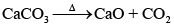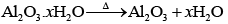Q.22. For the metallurgical process of which of the ores calcined ore can be reduced by carbon?
(i) Haematite
(ii) Calamine
(iii) Iron pyrites
(iv) Sphalerite
Ans. (i, ii)
Solution.
In the metallurgical process, the oxide ores are reduced by carbon. Sulphide ore cannot be reduced by carbon. Here haematite (Fe2O3) and calamine (ZnO) are oxide ores of iron and zinc respectively while iron pyrites (FeS2) and sphalerite (ZnS) are sulphide ores of iron and zinc respectively. Therefore, haematite and calamine can be reduced by carbon.

Q.23. The main reactions occurring in blast furnace during extraction of iron from haematite are ________.
(i) Fe2O+ 3CO → 2Fe + 3CO2
(ii) FeO + SiO2 → FeSiO3
(iii) Fe2O3 + 3C → 2Fe + 3CO
(iv) CaO + SiO2 → CaSiO3
Ans. (i, iv)
Solution.
In extraction of iron from haematite ore following reactions take place
(i) Fe2O+ 3CO → 2 Fe+ 3CO2
This reaction represents reduction of Fe2Oto Fe
(ii) CaO + SiO2 → CaSiO3

Q.24. In which of the following method of purification, metal is converted to its volatile compound which is decomposed to give pure metal?
(i) Heating with stream of carbon monoxide.
(ii) Heating with iodine.
(iii) Liquation.
(iv) Distillation.
Ans. (i, ii)
Solution.
In vapour phase refining method, the metal is converted into its volatile compound and collected elsewhere. It is then decomposed to give pure metal.
Vapour phase refining method includes
(i) Heating of metal with stream of CO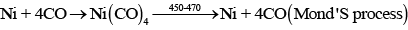(ii) Heating with iodine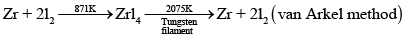Q.25. Which of the following statements are correct?
(i) A depressant prevents certain type of particle to come to the froth.
(ii) Copper matte contains Cu2S and ZnS.
(iii) The solidified copper obtained from reverberatory furnace has blistered appearance due to evolution of SO2 during the extraction.
(iv) Zinc can be extracted by self-reduction.
Ans. (i, iii)
Solution.
Correct statements are:
(i) A depressant prevents certain type of panicle to come to the froth, e.g. NaCN is added as a depressant during separation of PbS and ZnS.
(ii) The solidified copper obtained from reverberatory furnace has blistered appearance due to evolution of SO2 during the extraction.
(b) and (d) are incorrect statements, and can be correctly stated as
(iii) Copper matte contains Cu2S and FeS.
(iv) Zinc can be extracted by reduction of ZnO with carbon

Q.26. In the extraction of chlorine from brine _____________.
(i) ∆G° for the overall reaction is negative.
(ii) ∆G° for the overall reaction is positive.
(iii) E° for overall reaction has negative value.
(iv) E° for overall reaction has positive value.
Ans. (b, c)
Solution.
Electrolysis of brine solution is used to extract chlorine. Overall chemical reaction occurring in this process and value of ΔG° can be shown as
2H2O(l) + 2Cl(aq) → H2(g) + Cl2(g) + 2OH(aq)
For the given reaction, value of ΔG° is 422 kJ
Using ΔG° = -nFE°, the value of E° = -22V.
Therefore ΔG° for the overall reaction is positive and E° has negative value.

Q.27. Why is an external emf of more than 2.2V required for the extraction of Cl2 from brine?
Ans. Extraction of chlorine from brine (chlorine is abundant in sea water as common salt in the form of NaCl).
2Cl(aq) + 2H2O(l) → 2 OH(aq) + H2(g) + Cl2(g)
For the given reaction, value of ΔG° is + 422 kJ.
Using ΔDG° = -nFE°, the value of E° = -2.2 V
Therefore, an external emf greater than 2.2 V is required for the extraction of Cl2 from brine.

Q.28. At temperatures above 1073K coke can be used to reduce FeO to Fe. How can you justify this reduction with Ellingham diagram?
Ans. Using Ellingham diagram, we observe that at temperature greater than 1073 K; ΔG(C, CO) < DG (Fe, FeO). Now, we know that according to Ellingham diagram, compound having lower ΔrG° undergoes oxidation.
Hence, coke can reduce FeO to Fe.

Q.29. Wrought iron is the purest form of iron. Write a reaction used for the preparation of wrought iron from cast iron. How can the impurities of sulphur, silicon and phosphorus be removed from cast iron?
Ans. Wrong iron or malleable iron is the purest form of commercial iron and is prepared from cast iron by heating with haematite in a reverberatory furnace so that C gets converted into carbon dioxide. This haematite causes oxidation of carbon to carbon monoxide: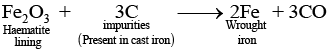Limestone is added as flux silicon, Sulphur and phosphorus are oxidised and passed into the slag. The metal is removed and freed from the slag by passing through rollers.

Q.30. How is copper extracted from low grade copper ores?

Ans. Copper is extracted from low grade copper ore using hydrometallurgy method. For this purpose, ore is leached out using bacteria. The solution containing Cu2+ is treated with scrap iron and H2.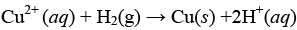Q.31. Write two basic requirements for refining of a metal by Mond's process and by Van Arkel Method.

Ans. Two basic requirements are:
(i) Metal should form volatile compound with available reagent.
(ii) The volatile compound should be easily decomposable so that it can be recovered easily
(a) Mond's process includes conversion of Ni to Ni (CO)4 and then decomposition of Ni(CO)4 to Ni.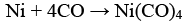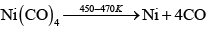(b) van Arkel method includes conversion of Zr to volatile ZrI4 and then decomposition of ZrI4 to Zr and I2.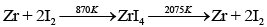Q.32. Although carbon and hydrogen are better reducing agents but they are not used to reduce metallic oxides at high temperatures. Why?

Ans. Because at high temperatures, carbon and hydrogen react with metals to form carbides and hydrides respectively. So, carbon and hydrogen are not better reducing agents to reduce metallic oxide.

Q.33. How do we separate two sulphide ores by Froth Floatation Method?  Explain with an example.
Ans. Two sulphide ores can be separated by adjusting the proportion of oil and water or by using depressants, e.g., if an ore contains ZnS and PbS both, a depressant NaCN is added during froth floatation. It forms a complex with ZnS and prevents it from coming into froth. PbS comes into the froth and thus separated.

Q.34. The purest form of iron is prepared by oxidising impurities from cast iron in a reverberatory furnace. Which iron ore is used to line the furnace? Explain by giving reaction.

Ans.
(a) Haematite (Fe2O3) ore is used to line the furnace
(b) The reaction involved

Q.35. The mixture of compounds A and B is passed through a column of Al2O3 by using alcohol as eluant. Compound A is eluted in preference to compound B.
Which of the compounds A or B, is more readily adsorbed on the column?
Ans. Compound which is adsorbed to the more extent comes out later while the other which absorbed to the less extent comes out readily. Since, compound A comes out before compound B, the compound B is more readily adsorbed on the column.

Q.36. Why is sulphide ore of copper heated in a furnace after mixing with silica?

Ans. Iron oxide present as impurity in sulphide ore of copper forms slag which is iron silicate and copper is produced in the form of copper matte.

Q.37. Why are sulphide ores converted to oxide before reduction?

Ans. This is because oxides are reduced to metals easily while sulphides are not, so, sulphide ores are converted to oxide before reduction.

Q.38. Which method is used for refining Zr and Ti? Explain with equation.

Ans. Zr and Ti are refined by van Arkel method. In this method crude metal is heated with iodine. Reactions involved are as follows:
(a) Formation of iodide: In case of zirconium
Zr+2I2 → ZrI4
(b) Decomposition of iodide: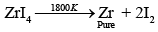Q.39. What should be the considerations during the extraction of metals by electrochemical method?

Ans. During extraction of metals by electrochemical method the following two points must be considered
(i) Reactivity of metals: If the metals are quite reactive and are expected to react with water then the metals should be extracted by the electrolysis of their purified molten ore rather than their aqueous solution.
(ii) Suitability of electrodes: The electrodes selected should not react with the product of electrolysis. If at all they react, then the electrodes must be made up of a cheap material so that their periodic replacement should not increase the cost of the process.

Q.40. What is the role of flux in metallurgical processes?
Ans. Role of flux in metallurgical process:
(i) Flux is used to remove the gangue by combining with it. Thus, slag formation takes place.
(ii) It makes the molten mass more conducting.

Q.41. How are metals used as semiconductors refined? What is the principle of the method used?
Ans. Semiconducting metal is produced by zone refining method which is based on the principle that the impurities are more soluble in molten state of metal than in solid state.

Q.42. Write down the reactions taking place in Blast furnace related to the metallurgy of iron in the temperature range 500-800 K.

Ans. Chemical reactions occurring in blast furnace related to the metallurgy of iron in the temperature range 500-800 K are
(i) 3Fe2O3 + CO → 2 Fe3O4 + CO2
(ii) Fe3O4 + 4CO → 3Fe + 4CO2
(iii) Fe2O3 + CO → 2FeO + CO2

Q.43. Give two requirements for vapour phase refining.

Ans. Two requirements for vapour phase refining are
(i) The metal should form a volatile compound with an available reagent.
(ii) The volatile compound should be easily recovered by decomposition.

Q.44. Write the chemical reactions involved in the extraction of gold by cyanide process. Also give the role of zinc in the extraction.

Ans. (i) 4Au(s) + 8CN(aq) + 2H2O (aq) + O2(g) →4 [Au(CN)2](aq) + 4OH(aq)
(ii) 2 [Au (CN)2](aq) + Zn(s) → 2Au(s) + [Zn(CN)4]2−(aq)
Zinc acts as a reducing agent in this reaction.

MATCHING TYPE

Note : Match the items given in Column I and Column II in the following questions.
Q.45. Match the items of Column I with items of Column II and assign the correct code: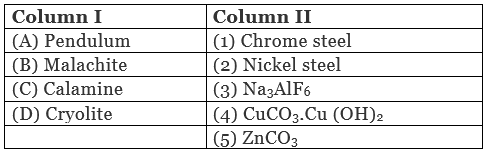Code : (i) A → (1) B → (2) C → (3) D → (4)
(ii) A → (2) B → (4) C → (5) D → (3)
(iii) A → (2) B → (3) C → (4) D → (5)
(iv) A → (4) B → (5) C → (3) D → (2)
Ans. (ii)
Solution.
A. Pendulum is made up of nickel steel.
B. Molecular formula of malachite is Cu CO3.Cu (OH)2.
C. Molecular formula of calamine is ZnCO3.
D. Molecular formula of cryolite isNa3AIF6.

Q.46. Match the items of Column I with the items of Column II and assign the correct code: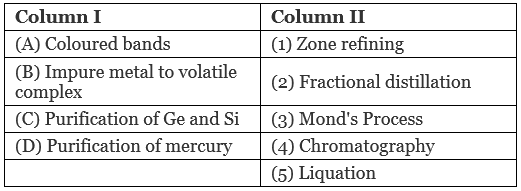Code:
(i) A → (1) B → (2) C → (4) D → (5)
(ii) A → (4) B → (3) C → (1) D → (2)
(iii) A → (3) B → (4) C → (2) D → (1)
(iv) A → (5) B → (4) C → (3) D → (2)

Ans. (ii)
Solution.
A. Coloured bands are observed in chromatography.
B. Impure metal is converted to volatile complex by using Mond's process.
C. Ge ans Si metals are purified by zone refining method.
D. Purification of mercury is done by fractional distillation.

Q.47. Match items of Column I with the items of Column II and assign the correct code: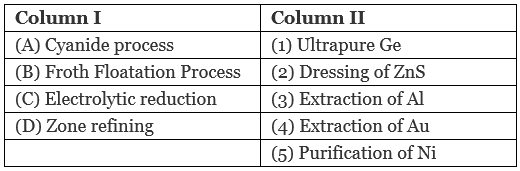Code:
(i) A → (4) B → (2) C → (3) D → (1)
(ii) A → (2) B → (3) → C (1) D → (5)
(iii) A → (1) B → (2) C → (3) D → (4)
(iv) A → (3) B → (4) C → (5) D → (1)

Ans. (i)
Solution.
A. Cyanide process is used for extraction of Au through formation of anionic complex [Au CN)2]
B. Froth floatation process is used for dressing of ZnS.
C. Electrolytic reduction method is used for extraction of aluminum. Graphite electrode is used for this purpose
D. Zone-refining is used for purification of Ge.

Q.48. Match the items of Column I with the items of Column II and assign the correct code: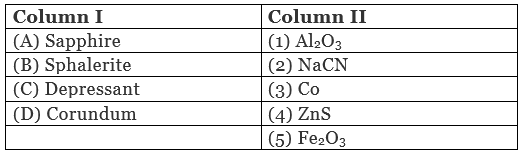Code:
(i) A → (3) B → (4) C → (2) D → (1)
(ii) A → (5) B → (4) C → (3) D → (2)
(iii) A → (2) B → (3) C → (4) D → (5)
(iv) A → (1) B → (2) C → (3) D → (4)

Ans. (i)
Solution.
A. Sapphire is a gemstone which contain Co.
B. Molecular formula of sphalerite is ZnS.
C. NaCN is used as a depressant in froth floatation method.
D. Molecular formula of corundum is Al2O3.

Q.49. Match the items of Column I with items of Column II and assign the correct code: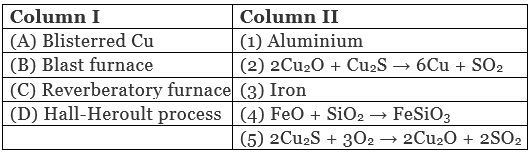Code:
(i) A → (2) B → (3) C → (4) D → (1)
(ii) A → (1) B → (2) C → (3) D → (5)
(iii) A → (5) B → (4) C → (3) D → (2)
(iv) A → (4) B → (5) C → (3) D → (2)

Ans. (i)
Solution.
A. Blisterred Cu can be prepared by means of following chemical reaction
2Cu2O + Cu2S → 6Cu + SO2
B. Iron is extracted by using blast furnace.
C. In reverberatory furnace formation of slag occurs as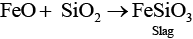D. Hall-Heroult process is used for extraction of aluminum.

ASSERTION AND REASON TYPE

Note : In the following questions a statement of Assertion followed by a statement of Reason is given. Choose the correct answer out of the following choices.
(i) Both assertion and reason are true and reason is the correct explanation of assertion.
(ii) Both assertion and reason are true but reason is not the correct explanation of assertion.
(iii) Assertion is true but reason is false.
(iv) Assertion is false but reason is true.
(v) Assertion and reason both are wrong.

Q.50. Assertion : Nickel can be purified by Mond process.
Reason : Ni (CO)4 is a volatile compound which decomposes at 460K to give pure Ni.
(i) Both assertion and reason are true and reason is the correct explanation of assertion.
(ii) Both assertion and reason are true but reason is not the correct explanation of assertion.
(iii) Assertion is true but reason is false.
(iv) Assertion is false but reason is true.
(v) Assertion and reason both are wrong.

Ans. (i)
Solution.

Nickel can be purified by Mond's process in which formation of a volatile compound Ni (CO)4 takes place which further decomposes to Ni at 460K.

Q.51. Assertion : Zirconium can be purificed by Van Arkel method.
Reason : ZrI4 is volatile and decomposes at 1800K.
(i) Both assertion and reason are true and reason is the correct explanation of assertion.
(ii) Both assertion and reason are true but reason is not the correct explanation of assertion.
(iii) Assertion is true but reason is false.
(iv) Assertion is false but reason is true.
(v) Assertion and reason both are wrong.

Ans. (i)
Solution.

Zirconium can be purified by van Arkel method which includes formation of volatile ZrI4 which decomposes at 1800 K to Zr.

Q.52. Assertion : Sulphide ores are concentrated by Froth Flotation method.
Reason : Cresols stabilise the froth in Froth Flotation method.
(i) Both assertion and reason are true and reason is the correct explanation of assertion.
(ii) Both assertion and reason are true but reason is not the correct explanation of assertion.
(iii) Assertion is true but reason is false.
(iv) Assertion is false but reason is true.
(v) Assertion and reason both are wrong.

Ans. (ii)
Solution.

Sulphide ores are concentrated by froth floatation method. Sulphide ore particles are preferentially wetted by oil, become lighter and rise to the surface along with the froth while gangue particles are preferentially wetted by water, become heavier and thus settle down at the bottom of the tank and cresols stabilise the froth in froth floatation method. Formation of froth is main reason for extraction of metal. Metal ore comes out along

Q.53. Assertion : Zone refining method is very useful for producing semiconductors.
Reason : Semiconductors are of high purity.
(i) Both assertion and reason are true and reason is the correct explanation of assertion.
(ii) Both assertion and reason are true but reason is not the correct explanation of assertion.
(iii) Assertion is true but reason is false.
(iv) Assertion is false but reason is true.
(v) Assertion and reason both are wrong.

Ans. (ii)
Solution.

Zone refining method is very useful for producing semiconductors of high purity as in this process pure metal crystallises while impurities pass on into adjacent molten zone when impure metal rod is heated.

Q.54. Assertion : Hydrometallurgy involves dissolving the ore in a suitable reagent followed by precipitation by a more electropositive metal.
Reason : Copper is extracted by hydrometallurgy.
(i) Both assertion and reason are true and reason is the correct explanation of assertion.
(ii) Both assertion and reason are true but reason is not the correct explanation of assertion.
(iii) Assertion is true but reason is false.
(iv) Assertion is false but reason is true.
(v) Assertion and reason both are wrong.

Ans. (ii)
Solution.

Hydrometallurgy involves dissolving the ore in suitable reagent followed by precipitation with the help of more electropositive metal in which pure metal is replaced by more electropositive metal.

Q.55. Explain the following :
(a) CO2 is a better reducing agent below 710K whereas CO is a better reducing agent above 710K.
(b) Generally sulphide ores are converted into oxides before reduction.
(c) Silica is added to the sulphide ore of copper in the reverberatory furnace.
(d) Carbon and hydrogen are not used as reducing agents at high temperatures.
(e) Vapour phase refining method is used for the purification of Ti.
Ans. (a) As shown in Ellingham diagram which relates Gibbs free energy and temperature at below 710 K, we have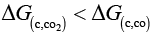So, CO2 is a better reducing agent than CO while above 710K it becomes a very good reducing agent.
(b) Generally, sulphide ores are converted to oxides before reduction as reduction of oxides can easily be done using C or CO depending upon metal ore and temperature.
(c) Silica is a flux added to the sulphide ore of copper in the reverberatory furnace leading to the formation of slag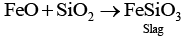(d) Carbon and hydrogen are not used as reducing agents at high temperature. At high temperature carbon and hydrogen readily form their carbides and hydrides respectively.
(e) Vapour phase refining method is used for the purification of Ti as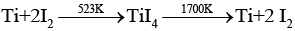Offer running on EduRev: Apply code STAYHOME200 to get INR 200 off on our premium plan EduRev Infinity!

233 docs

,

,

,

,

,

,

,

,

,

,

,

,

,

,

,

,

,

,

,

,

,

;